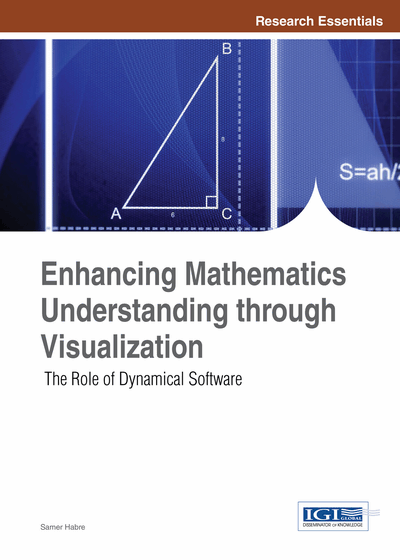# String Art and Linear Iterative Systems

Samer Habre (Lebanese American University, Lebanon)
DOI: 10.4018/978-1-4666-4050-4.ch012
Available
\$37.50
No Current Special Offers

## Abstract

String art dates back to the 19th century when it was initially invented to ease the delivery of some mathematical ideas. Since then, the art has evolved and so has its use in mathematics. In this chapter, the authors see how some of the phase portraits for 2 x 2 linear homogeneous iterative systems exhibit some artistic behavior that resembles this form of art. The investigation gives a sufficient condition for the solutions of such systems to form closed cycles. However, in other situations the cycles formed are infinite, producing some fascinating examples of string art.
Chapter Preview
Top

## Introduction

String art has its origins in the “curve stitch” activities invented by Mary Everest Boole (1832–1916) at the end of the 19th Century. String art or “mathematical embroidery” is a family of lines created by attaching strings to artfully located sets of pins forming abstract geometric patterns (Pedoe, 1976). Even though straight lines are formed by the string, yet the eyes of the observer pick a phantom curve or an “envelope”. Mathematically, the envelope of a one parameter family of lines is the curve which is mutually tangent to that family (Struik, 1950). In simpler terms, strings can be woven so as to envelope various geometric figures such as a polygon (Figure 1a), the shape of a hyperbola (Figure 1b), or simply some beautiful design (Figure 1c).

String art was initiated by Mary Boole to make mathematical ideas more accessible to children. Boole, the wife of mathematician George Boole, was a self-taught mathematician; she wrote many didactic works on mathematics, such as The Preparation of the Child for Science (Boole, 1904), and Philosophy and Fun of Algebra (Boole, 1909). The latter book encouraged children to explore mathematics through playful activities such as “curve stitching”.

In the 20th century, this art was popularized as a decorative craft. There are however many instances where curve stitching is used for elementary and middle school mathematics. In more elaborate instances, string art has been used to introduce more advanced mathematical ideas such as Bézier curves. In this chapter, I present to the reader yet another mathematical instance that is associated with this form of art. The solutions of linear iterative systems as introduced in Chapter 4 of this book and when sketched using the dynamical software developed by Hu Hohn can exhibit some fascinating figures that may be classified as a form of string art.

Top

## Linear Systems Of Iterative Equations And String Art

Linear iterative systems were introduced and discussed in Chapter 4. These take the form:

To understand such systems is to understand the fate of the iteration when starting with an arbitrary point in the plane (initial conditions). In all cases, the point (0,0) is an equilibrium solution in the sense that the iterated values will remain unchanged. A complete study of such systems was done by the author and it appeared in a paper entitled “A Complete Classification ofLinear Iterative Systems” (Habre & McDill, 2008). It was found that the shape and long term behavior of the solutions to the iterative systems depend on the number of eigenvalues of the system matrixAs mentioned in Chapter 4, Hu Hohn from the Massachusetts College of Arts developed the dynamical tool Linear Iterated Systems to visualize the fate of any iteration. We give an example.

## Complete Chapter List

Search this Book:
Reset